# University of Wisconsin Green Bay

A model rocket is launched straight up into the air from ground level. The rocket engine burns for 3.2 s and provides a net upward acceleration during that time period of 27 m/s2. How high does the rocket rise before it starts down again?

• In this problem, you are asked to describe the motion (how high) of the rocket. Whenever you are asked to describe the motion of an object without worrying about the cause of that motion, you have a kinematics problem.

•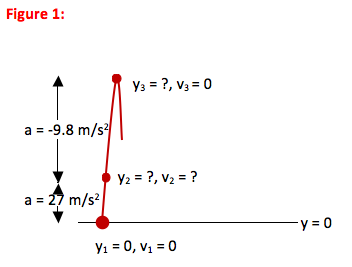•There are three key kinematic equations. If you carefully select the equation which most directly describes the situation in your problem, you will not only solve the problem in fewer steps but also understand it better. The three equations, written for motion in the y-direction, are:

1. y = y0 + v0Δt + ½ a(Δt)2 (relates position and time)

2. v = v0 + aΔt (relates velocity and time)

3. v2 = v02 + 2a(Δy) (relates velocity and position)

These equations only apply for periods of motion in which acceleration is constant! In this problem, the acceleration of the rocket changes from one constant value to another at Point 2. Therefore, you will have to work the problem in two parts.

a) To find the distance the rocket rises in the first 3.2 seconds (from Point 1 to Point 2), relate position and time: equation 1 with a = 27 m/s2.

b) To find the distance the rocket rises between Point 2 and its maximum height (y-velocity = 0), relate position and velocity: equation 3 with a = -9.8 m/s2. (You might also recognize that to use equation 3 you will need to know the velocity at Point 2, or the velocity at 3.2 seconds. This requires equation 2 between Points 2 and 3. If you don’t catch that at this stage, that is fine. You will recognize it as you work the problem.)

• Step 1: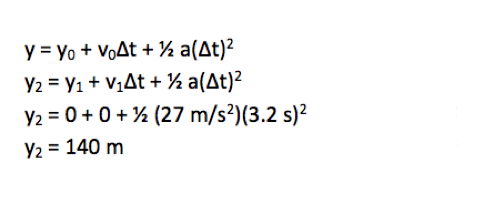You now know how high the rocket flew during the period of time while the engine was burning. Scroll down to find how much further the rocket went before coming to a stop at the top of the motion.

------------------------------------------------------------------------

Step 2:

Now that you know how high the rocket flies while the engine burns, you need to find how much further it goes to reach its maximum height: in other words, the distance between Point 2 and Point 3. All equations that relate Points 2 and 3 require you to know the velocity of the rocket at Point 2, so this must be done as an intermediate step.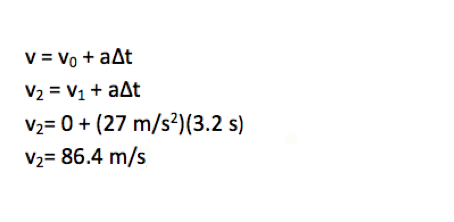You now have the information needed to solve kinematic equations for Point 3. Scroll down to continue with the solution.

------------------------------------------------------------------------

Step 3: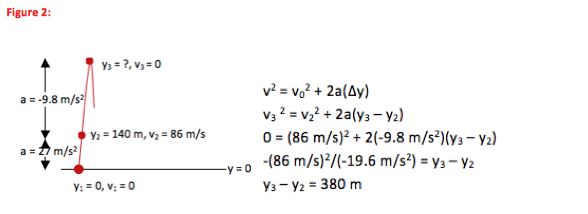The rocket travels upward for 140 m between Points 1 and 2, and an additional 380 m between Points 2 and 3, so it travels a total of (140 m + 380 m) = 520 m to its highest point.

•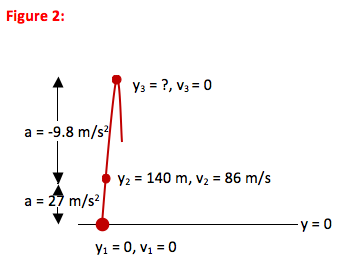The rocket in this problem experienced a change in acceleration when the engine stopped burning. The kinematic equations are only valid for periods of constant acceleration. (They were derived from the definitions of velocity and acceleration plus the condition of constant acceleration.) Therefore, you had to work the problem in two parts.

During the first part of the problem, the observed acceleration of the rocket was 27 m/s2 and the rocket accelerated from zero to 86 m/s while traveling a distance of 140 m. Knowing these values at Point 2 allowed you to then learn about Point 3, with the understanding that the observed acceleration of the rocket between Points 2 and 3 was -9.8 m/s2.

Because the initial acceleration is almost a factor of 3 greater than the final acceleration, it makes sense that the distance covered in the second part of the motion is almost a factor of three greater than in the initial leg. In both cases, the rocket went between speeds of 0 and 86 m/s, but it took a much longer time to do so after the engine shut off.